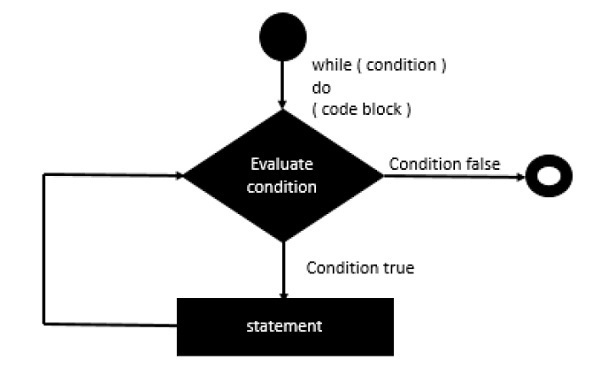# Clojure - While Statement

## Syntax

Following is the syntax of the ‘while’ statement.

```(while(expression)
(do
codeblock))
```

The while statement is executed by first evaluating the condition expression (a Boolean value), and if the result is true, then the statements in the while loop are executed. The process is repeated starting from the evaluation of the condition in the while statement. This loop continues until the condition evaluates to false. When the condition is false, the loop terminates. The program logic then continues with the statement immediately following the while statement. Following is the diagrammatic representation of this loop.## Example

Following is an example of a while loop statement.

```(ns clojure.examples.hello
(:gen-class))

;; This program displays Hello World
(defn Example []
(def x (atom 1))
(while ( < @x 5 )
(do
(println @x)
(swap! x inc))))
(Example)
```

In the above example, we are first initializing the value of ‘x’ variable to 1. Note that we are using an atom value, which is a value which can be modified. Then our condition in the while loop is that we are evaluating the condition of the expression to be such as ‘x’ should be less than 5. Till the value of ‘x’ is less than 5, we will print the value of ‘x’ and then increase its value. The swap statement is used to populate the atom variable of ‘x’ with the new incremented value.

## Output

The above code produces the following output.

```1
2
3
4
```
clojure_loops.htm